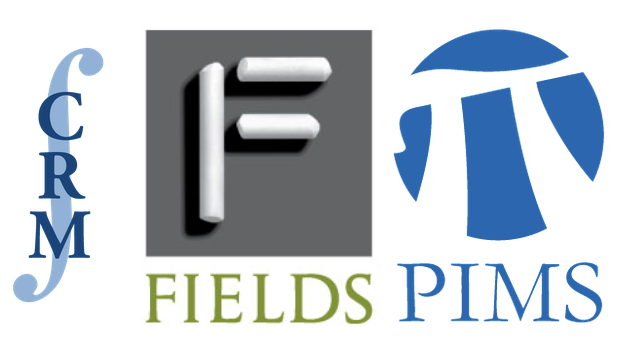# The 2018 CRM-Fields-PIMS Prize is awarded to Jeremy QuastelThe Centre de recherches mathématiques (CRM), the Fields Institute, and the Pacific Institute for the Mathematical Sciences (PIMS) are proud to award the 2018 CRM-Fields-PIMS Prize to Jeremy Quastel (University of Toronto).

Jeremy Quastel is widely recognized as one of the top probabilists in the world, having made major advances in the fields of hydrodynamic theory, stochastic partial differential equations, and integrable probability. He is particularly recognized for a series of ground-breaking works during the last ten years related to the Kardar-Parisi-Zhang (KPZ) equation and the wider class of random growth models conjectured to share the same long-time, large-scale limit (the so-called KPZ universality class). He proved a 25 year old conjecture from physics about the scaling exponents for the KPZ equation, as well as computing an exact formula for its one-point distribution. He demonstrated that the KPZ equation is universal in that it arises as a scaling limit of a wide variety of non-linear stochastic partial differential equations of Hamilton-Jacobi type. Most recently, he constructed and computed transition probabilities for the ‘KPZ fixed point’ Markov process, which should be the universal long-time limit of all models in the KPZ universality class. Among his earlier contributions, Quastel derived the incompressible Navier-Stokes equation from a class of interacting particle systems, derived equations for the behaviour of the internal diffusion-limited-aggregation model, and proved a conjecture about the speed of the traveling front for the stochastic Fisher-Kolmogorov-Petrovsky-Piskunov equation, which models branching diffusion processes.

For the profound impact of his work, Quastel has been recognized as a Fellow of the Royal Society of Canada (2016), and was the recipient of a Killam Research Fellowship (2013). He delivered an invited address at the 2010 International Congress of Mathematicians in Hyderabad India.

Jeremy Quastel received his Ph.D. from the Courant Institute in 1990. After six years at the University of California, Davis, he moved to his present position at the University of Toronto in 1998.

Conference slideshow

Conference video